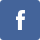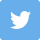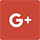# Free 11 Plus Bidmas Practice Papers

Free 11 Plus Bidmas Practice Papers

Yes! You have read it correctly. There are Free 11 Plus Bidmas Practice Papers available in 11PluseHelp.co.uk.

We have Free 11 Plus BIDMAS practice Papers at 11PluseHelp.co.uk.

BIDMAS

BIDMAS explains the order of operations to solve an expression containing all the basic operations. It is abbreviated as Brackets, Indices, Division, Multiplication, Addition, and Subtraction. First, need to solve the bracket( (), {}, []) followed by powers and roots, division, multiplication, addition and subtraction from left to right.

Example:

Can you tell the answer of ( 5 + 6 ) × 4?

( 5 + 6 ) × 4

First Step:
In the first step, we should solve the bracket according to the BIDMAS rule.

After solving the bracket, we get the answer 11.

11 x 4

Second Step:
Now, we have to multiply 11 and 4. After multiplication, we get the answer 44.

44

Mathematics is a logic-based discipline. There are some simple rules to follow, which will help you work out to do the sum. This rule is called as BIDMAS rule. This rule was introduced by Achilles Reselfelt as early as 1600. The main aim of introducing this kind of mathematical rule was to solve those problems which involve more than one mathematical operation in a mathematical problem.

BIDMAS is an acronym. Using this specific rule makes it easy for us to keep in mind the order of the operations while solving different kinds of mathematical problems or operations.

The meanings of different words of this acronym are given below;1. B — Bracket

It means that first of all, we have to solve the brackets in the mathematical operation.

There are four different kinds of brackets.

First of all, we have to solve ━━━━”.

Secondly, we have to solve ().

Thirdly, we have to solve {}.

At last, we have to solve [].

1. I — Indices

It means that you will have to solve all the numbers which have powers and brackets.

1. D — Division

It means that you have to perform the division operation.

1. M — Multiplication

After that, you have to perform the multiplication operation.

After that, you have to add the numbers or terms.

1. S — Subtraction

At last, you have to subtract the numbers and terms.

Let’s see some examples of usage of the BIDMAS rule:

Example 1

4[2 + { 7(5-3)}]

First Step

In the first step, we have to solve (). After solving it, we get:

4 [ 2 + { 7 x 2 } ]

Second Step

Now, we have to solve { }. After solving it, we get:

4 [ 2 + 14 ]

Third Step

Now, we have to solve [ ]. After solving it, we get:

4 x16

At last, we have to multiply these two terms. After multiplying 4 and 16, we get the answer 64.

64

Example 2

Solve 20 x 2 – ( 4 / 2 ) x 9 x 2^2

(Here 2^2 is square of ‘2’)

First Step

20 x 2 – ( 4 / 2 ) x 9 x 2^2

Second Step

20 x 2 – 2 x 9 x 2^2

Third Step

20 x 2 – 2 x 9 x 4

Fourth Step

20 x 2 – 72

Fifth Step

40 – 72

Sixth Step

-32

By following this specific rule, you will be able to easily and perfectly solve any mathematical operation. We have plenty of 11 Plus Practice tests on BIDMAS at 11PluseHelp.co.uk

You can access 11 Plus Practice Papers on different topics here:

https://www.11plusehelp.co.uk/11-plus-practice-papers

Examples from our 11 plus practice tests are given below:

Example1:

Solve 3 x 7+ (9-4)-6=?

Step1: Brackets: 3 x 7 + (5) – 6

Step2: Multiplication: 21+ (5) – 6

Example2:

Solve 2 x 5 – (1+4) ÷ 5=?

Step1: Brackets: 2 x 5-(5) ÷ 5

Step2: Division: 2 x 5 -1

Step3: Multiplication: 10-1

Step4: Subtraction: 10-1 = 9

Example3:

Solve 9 x (10-6) + 4=?

Step1: Brackets: 9 x 4 + 4

Step2: Multiplication: 36 + 4

Example4:

Solve 2 x 5-(1+4)/5=?

Step1: Brackets: 2 x 5-(5)/5

Step2: Multiplication: 10-1

Step3: Subtraction: 10-1=9

You can access 11 plus maths papers on different topics here:

https://www.11plusehelp.co.uk/11-plus-maths-practice-tests

11PluseHelp.co.uk is a one-stop-shop for 11 Plus exam preparation, including 11 Plus Independent schools preparation.

We focus on fundamentals, logic, basics and cover most of the syllabus for all 11 Plus exam patterns in the UK. For example, We cover Maths topic wise questions and fundamentals and how to apply them. We have constantly been adding 11 Plus practice papers regularly.

Free 11 Plus Area and Perimeter Practice Papers:

Free 11 Plus Area and Perimeter Practice Papers

11plusehelp.co.uk provides different types of problems on each 11 plus maths topics with 11 Plus Answer sheets. It provides maths past papers in the form of multiple-choice or blank type practice paper with time-bound. We provide word problems on maths topics like addition subtraction, skip counting, age range, pre-algebra, fraction decimals, etc.11plusehelp.co.uk is useful to increase students’ math skills. We provided flashcards to revise the subjects.

Free 11 Plus Maths Topic – Wise Practice Papers:

Free 11 Plus Maths Topic-wise Practice Papers

Free 11 Plus English Topic – Wise Practice Papers:

Free 11 Plus English Topic-wise Practice Papers

Free 11 Plus Verbal Reasoning Topic – Wise Practice Papers:

Free 11 Plus Verbal Reasoning Topic-wise Practice Papers

Free 11 Plus Non-Verbal Reasoning Topic – Wise Practice Papers:

Free 11 Plus Non-Verbal Reasoning Topic-wise Practice Papers

We have loads of questions useful for preparing CEM, CSSE and GL assessment and any other 11 Plus entrance exams in the UK. Children will love to take these tests, and learning is fun at 11plusehelp.co.uk.

https://www.11plusehelp.co.uk/list-of-grammar-schools

https://www.11plusehelp.co.uk/top-100-independent-schools

At 11plusehelp.co.uk/, we possess the following resources:

Maths11 Plus Maths Worksheets, 11 Plus Maths Papers free download, 11 Plus Maths topics pdfs.

English11 Plus English papers with answers, 11 Plus comprehension with answers, 11 English comprehensions multiple-choice, English reasoning test.

https://www.11plusehelp.co.uk/11-plus-topics/11-plus-english-topics

Verbal and Non-Verbal Reasoning11 Plus Verbal Reasoning worksheets free, Verbal and Non-Verbal Reasoning tests, easy Non-Verbal Reasoning Worksheets

You can access 11 Plus Verbal Reasoning Practice Papers on different topics here:

https://www.11plusehelp.co.uk/11-plus-practice-papers/11-plus-english-practice-test-papers/11-plus-verbal-practice-papers

You can access 11 Plus Non-Verbal Reasoning Practice Papers on different topics here:

https://www.11plusehelp.co.uk/11-plus-practice-papers/11-plus-english-practice-test-papers/11-plus-non-verbal-reasoning-practice-tests

Practice TestsEleven plus sample papers, free 11 plus papers with answers, 11 plus mock test papers pdf, 11 exam question and answers, UK 11 plus exam questions, 11 online tests in Maths and English with 11 Plus answers.

11 Plus Revision Pack:

https://www.11plusehelp.co.uk/11-plus-practice-papers/11-plus-english-practice-test-papers/revision-pack

To access Virtual Mock Exams, please visit :

https://11plus-mockexams.co.uk/

You can access 11 Plus FREE Papers by visiting the below link:
https://www.11plusehelp.co.uk/11-plus-free-online-papers

You can access 11 Plus FREE Sample Papers by visiting the below link:
https://www.11plusehelp.co.uk/11-plus-sample-papers

11 Plus complete solution features:

https://11plusehelp.co.uk/blog/2017/05/01/11-plus-complete-solution/

Practice and Perseverance Over Genius and Talent

Thanks,

11Plusehelp.co.uk